# Chapter # 02 : Program and Algorithm

### What is a Program?

program is a sequence of instructions (called programming statements), executing one after another - usually in a sequential manner, as illustrated in the following flow chart Figure 1.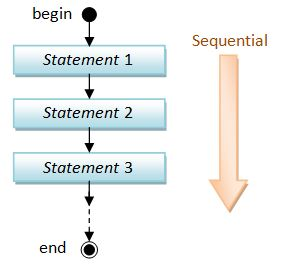Figure 1​​ Flow Chart

### What is Algorithm?

An algorithm is a finite set of instructions or logic, written in order, to accomplish a certain predefined task. Algorithm is not the complete code or program, it is just the core logic (solution) of a problem, which can be expressed either as an informal high level description as pseudocode or using a flowchart

### Steps in the development of Algorithm?

• Problem definition

• Development of a model

• Specification of Algorithm

• Designing an Algorithm

• Checking the correctness of Algorithm

• Analysis of Algorithm

• Implementation of Algorithm

• Program testing

• Documentation Preparation

Every Algorithm must satisfy the following properties

• Input- There should be 0 or more inputs supplied externally to the algorithm.

• Output- There should be at least 1 output obtained.

• Definiteness- Every step of the algorithm should be clear and well defined.

• Finiteness- The algorithm should have finite number of steps.

• Correctness- Every step of the algorithm must generate a correct output.

** Don’t miss the Key Requirements

### What are the symbols used Algorithm?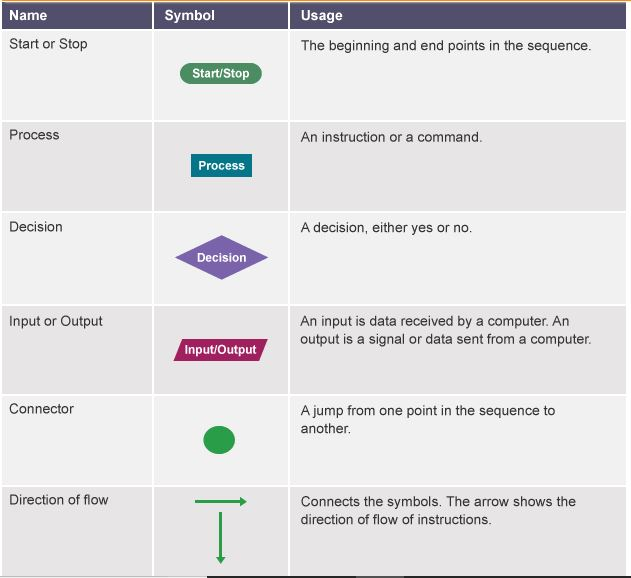Figure 2​​ Following Symbols we can user in making Algorithms

###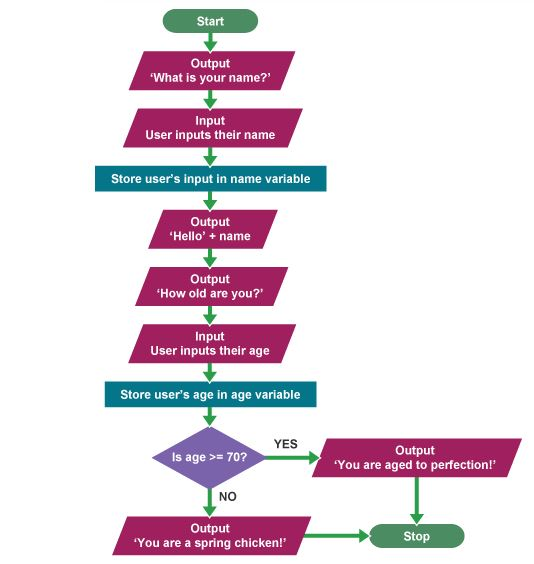Figure 3​​ Example of representation

### If-Then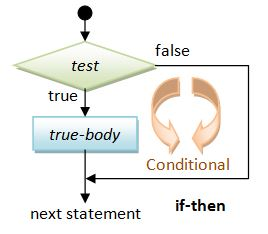### If-Then-else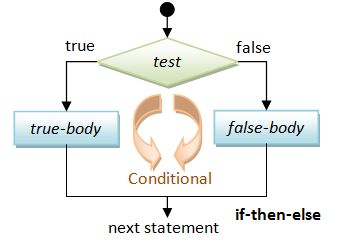### Loop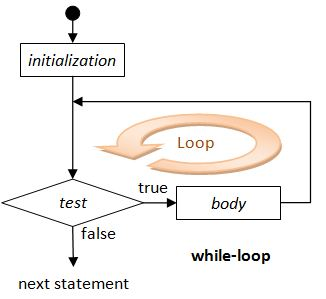### Basic Arithmetic Operations

 Operator Meaning Example + Addition x + y - Subtraction x - y * Multiplication x * y / Division x / y % Modulus (Remainder) x % y ++ Increment by 1 (Unary) ++x or x++ -- Decrement by 1 (Unary) --x or x-- && Logical AND (x >= 1) && (x <= 100) || Logical OR (x < 1) || (x > 100) ! Logical NOT !(x == 8)